# Division - 8th grade (13y) - math problems

#### Number of problems found: 182

• Factory and divisionsThe factory consists of three auxiliary divisions total 2,406 employees. The second division has 76 employees less than 1st division and 3rd division has 212 employees more than the 2nd. How many employees has each division?
• RemainderA is an arbitrary integer that gives remainder 1 in the division with 6. B is an arbitrary integer that gives remainder 2 the division by. What makes remainder in division by 3 product of numbers A x B ?
• Reciprocal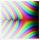What is the reciprocal of the sum of the reciprocals of 4 and 9?
• Sum of dividendIf X is divided separately 2.63 and 1.40. And the results of the individual divisions are added to give a result less than X. What is X?
• Numbers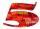Write smallest three-digit number, which in division 5 and 7 gives the rest 2.
• Ratio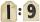Unfold number 1963 in the ratio 9:2:2.
• Find the 11Find the quotient of 229.12 and 12.32
• DivisibilityWrite all the integers x divisible by seven and eight at the same time for which the following applies: 100
• Number ratio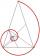Calculate two positive numbers that its ratio is 6:6 and difference was 0.
• Two numbersThe difference between the two numbers is 74. If we divide a larger number by a smaller one, we get a quotient 7 and the rest of 2. Determine both numbers.
• Plum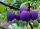On the platter are plum. How many were there if its have to be able to share equally among 8,10 and 12 children?
• Divisors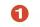Find all divisors of number 493. How many are them?
• ArcConvert to arc measures (radians): 924 ° Result write as a multiple of π
• An airplaneAn airplane flies 1440 km in 2 1/4 hours. What is its average speed in km per hour?
• Salary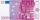Lawyer got to pay 840 Euros in banknotes of 20 and 50 Eur. Total got 18 banknotes. How many was which?
• Percent calculationCalculate 51% if 99% is 79.
• Euros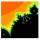Michal, Peter, John and Lenka got together 2,400 euros. They share an amount in ratio 2:6:4:3. How many got each of them?
• Divide 5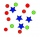Divide 288 in the following ratio 3 : 4 : 5
• 7 digit numberIf 3c54d10 is divisible by 330, what is the sum of c and d?
• Screws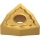The box contains 8 iron, 6 brass, and 4 titanium screws. What is the probability that the randomly selected screw will not be brass?

Do you have an interesting mathematical word problem that you can't solve it? Submit a math problem, and we can try to solve it.

We will send a solution to your e-mail address. Solved examples are also published here. Please enter the e-mail correctly and check whether you don't have a full mailbox.

Please do not submit problems from current active competitions such as Mathematical Olympiad, correspondence seminars etc...